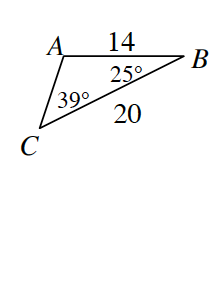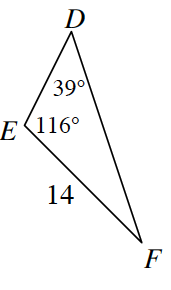Home > CCG > Chapter Ch7 > Lesson 7.1.1 > Problem7-6

7-6.

Examine $ΔABC$ and $ΔDEF$ at right.

1. Assume the triangles above are not drawn to scale. Complete a flowchart to justify the relationship between the two triangles.

Use the Triangle Angle Sum Theorem to find the unknown angle in each triangle.
2. Find $AC$ and $DF$.